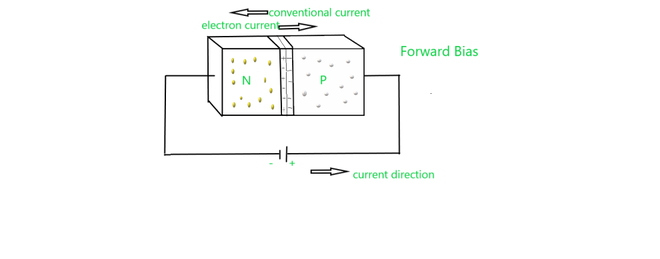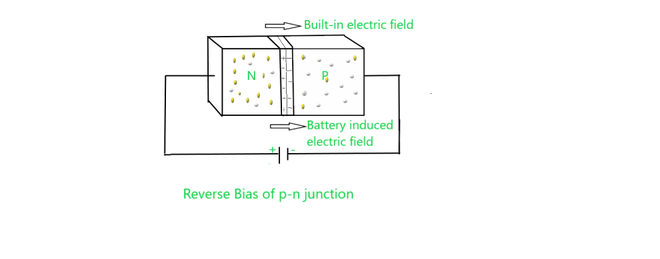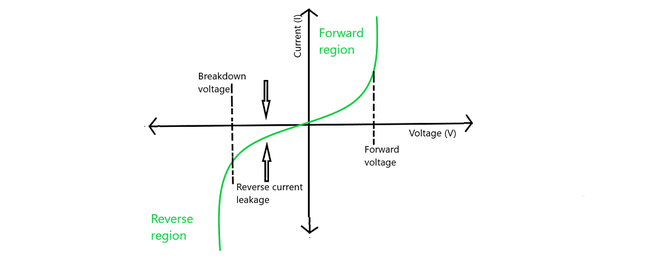GeeksforGeeks App
Open AppBrowser
Continue

# VI Characteristics of a P-N Junction Diode

Semiconductors is a kind of material that has resistivity and conductivity in between metals and insulators. On the basis of purity semiconductors are of two types: Intrinsic Semiconductors is a kind of pure semiconductor without any significant dopant (impurities) species present. An intrinsic semiconductor is also called an undoped semiconductor and Extrinsic Semiconductors is a kind of semiconductor they are doped with an impurity, it is known as an extrinsic semiconductor.

### p-n Junction Diode

Junction generally means the area or point that bounds two different parts, similarly in diodes junction is a boundary of two semiconductor materials i.e. the p-type and the n-type, semiconductor.

The p-side in the p-n junction has a positive side of the semiconductor and it has an excess of holes whereas the n-side has an excess of electrons, therefore, it is the negative side. The p-n junction in semiconductors is developed by the method of doping. adding impurities in a semiconductor is known as doping.

### Formation of p-n Junction

Let us understand for an example, consider a thin p-type silicon semiconductor sheet. If we add a small amount of pentavalent impurity(having valency five) to this, a part of the p-type Si will get converted to n-type silicon. This sheet will now contain both regions i.e. p and n-type region and a junction is created between two regions.

• There are two types of processes that follow after the formation of a p-n junction – diffusion and drift. As we know, diffusion is the process that follows the flow of particles from higher concentration to lower concentration, due to difference in the concentration of electrons and holes at the two sides of a junction, the electrons from the n-side diffuse to the p-side and the holes from the p-side diffuse to the n-side. this leads to raise in diffusion current.
• Also, there is an ionized donor is left behind on the n-side, which is an immobile charge this develop when an electron diffuses from the n-side to the p-side. As the result of this process, a layer of positive charge is developed on the n-side of the junction.
• Similarly, An ionized acceptor is left behind in the p-side when a hole goes from the p-side to the n-side, resulting in the formation of a layer of negative charges in the p-side of the junction. This region of negative (-) and positive charge (+) on either side of the junction is termed the depletion region.
• An electric field direction from a positive charge towards the negative charge is developed, Due to this positive charge region on either side of the junction, Due to this electric field, the flow of electrons and holes takes place. This is termed the drift motion. generally, the direction of the drift current is opposite to that of the diffusion current.

Forward BiasThe forward bias of p-n  junction

In biasing semiconductor is connected to an external source. when the p-type semiconductor is connected to the positive terminal of the source or battery and negative terminal to the n-type, then this type of junction is said to be forward-biased. In forward bias the direction of built-in electric field near the junction and applied electric field are opposite in direction. this means that the resultant electric field has a magnitude lesser than the built-in electric field. due to this there is less resistivity and therefore depletion region is thinner. In silicon, at the voltage of 0.6 V, the resistance of the depletion region becomes completely negligible.

Reverse BiasThe reverse bias of p-n  junction

In the reverse biasing, the n-type is connected to the positive terminal and the p-type is connected to the negative terminal of the battery . In this case, the applied electric field and the built-in electric field are in the same direction and the resultant of electric field has higher magnitude than the built-in electric field creating a more resistive, therefore depletion region is thicker. if the applied voltage becomes larger, then the depletion region becomes more resistive and thicker.

p-n Junction Formula

The potential difference created by the electric field in the p-n junction is given by:

Eo = VT ln [Nd Na / ni2

where

• Eo  junction voltage at no bias,
• VT is the thermal voltage at room temperature i.e. 26mv,
• Nd and Na are the concentrations of impurity and
• ni is intrinsic concentration.

### V-I Characteristics of p-n Junction DiodeV-I characteristics of p-n junction diode

• In forward bias condition p-type is connected to positive terminal of battery and the n-type to the negative terminal of the battery, there is a reduction in the potential barrier, in this condition. For germanium diodes, when the voltage is 0.3 V, and for silicone diodes, when the voltage is 0.7 V the potential barriers decrease and there is a flow of current.
• When the diode is in forward bias, as the voltage applied to the diode is overcoming the potential barrier, the current increases slowly and the curve obtained is non-linear. Once the potential barrier is crossed by the diode, the diode behaves normally and the curve rises sharply as further external voltage increases and the curve obtained is linear.
• When the PN junction diode is under reverse bias, this results in an increase in the potential barrier and resistance also increases. Minority carriers are present in the junction which creates reverse saturation current flows in the beginning.
• If the applied voltage increases rapidly, there is increased kinetic energy due to minority charge carriers which affect the majority charges. In this stage the diode breaks down. or the voltage is called breakdown voltage, This may also destroy the diode.

### Sample Questions

Question 1: When silicon is doped with indium it leads to which type of semiconductor?

As we know, Valency of Indium is 3 therefore it is Trivalent in nature, when it is doped in Silicon it has majority of holes, that’s why it is of p-type semiconductor.

Question 2: A transistor has a current gain of 30 Ampere. If the collector resistance is 6 kΩ, the input resistance is 1 kΩ, calculate its voltage gain.

Given,

Rin =1 kΩ  and Rout = 6k Ω

∴ Rgain =  Rout/Rin  =  6/1 = 6

Voltage gain = current gain × Resistance gain

= 30 × 6 =180

Question 3: Write characteristics of holes.

Following are the characteristics of holes:

• A hole is equivalent to a positive electric charge.
• The mobility of a hole as compare to that of an electron is less.

Question 4: Name the kind of biasing which leads the following result:

a) Increase in resistance,

b) Decrease in resistance and

c) Increase in width of the depletion region.

a) In reverse bias resistance increases.

b) In forward bias resistance decrease.

c) In reverse bias there is increase in the width of depletion region take place.

Question 5: What is the ratio of electrons and holes in the intrinsic semiconductor?

Number of electrons = ne

Number of holes = nh

In intrinsic semiconductor, ne = nh

ne/nh =

Question 6: Define the term breakdown voltage of p-n junction.